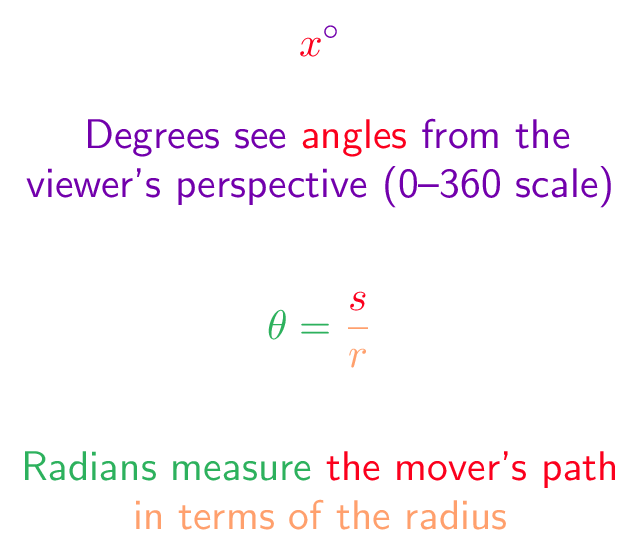## # Colorized Definition\newcommand{\degree}{\color{c1}}
\newcommand{\anglecolor}{\color{c3}}
\newcommand{\degreemeasure}{\color{c4}}
$$\degree \anglecolor{x}^{\degree{ \circ }}$$
$$\radian \theta = \anglecolor \radius \frac{\anglecolor s}{r}$$